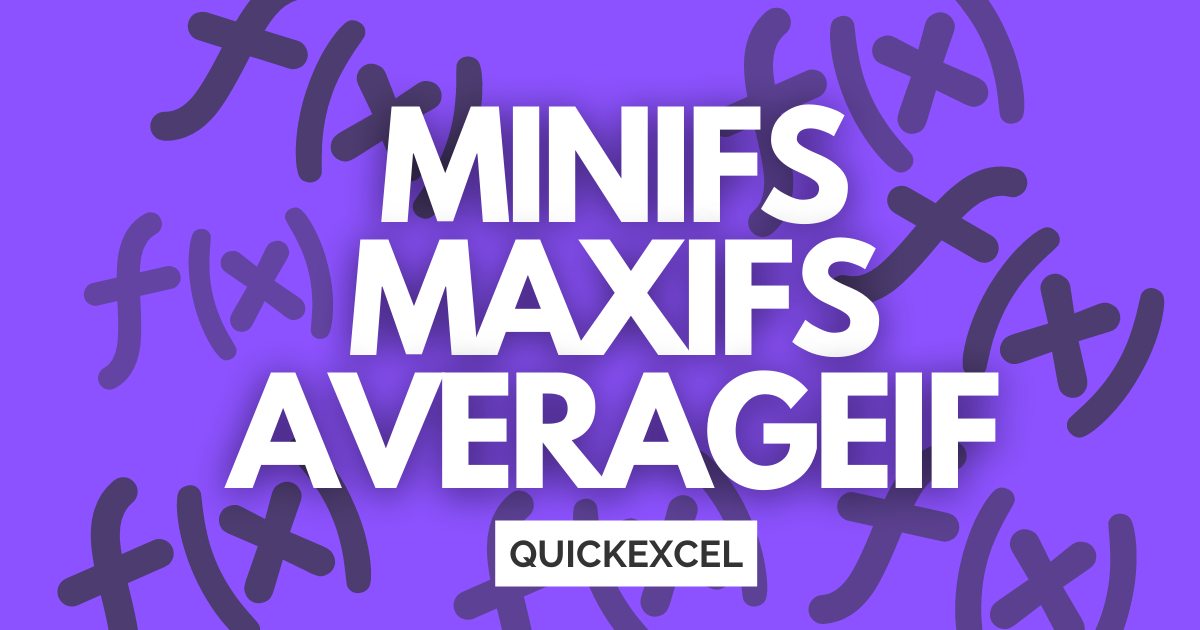# MINIFS, MAXIFS, and AVERAGEIF Formulas in ExcelIn this tutorial, we are going to learn about the very new MINIFS and MAXIFS formulas in Microsoft Excel. A lot of people are unaware of these formulas as it is only available for users using Excel 2016 or higher. Lastly, we will learn about the AVERAGEIF formula available in all versions of Excel.

Assuming that you do not live on a rock, you might as well be using the latest version of Excel and have these formulas built-in.

## What are the MAXIFS and MINIFS formulas?

These brand-new formulas work very similarly to the SUMIFS and COUNTIFS formula in Excel. They help you find the minimum or the maximum values from a range given set of conditions or criteria.

For example, you can find out the minimum or the maximum number of items purchased by a specific customer in a month, or the minimum or maximum revenue generated from a specific customer.

Let’s understand this with a concrete example.

### Steps to use the MINIFS formula

Here is a small list of customers of a company and the total revenue earned by them. Our goal is to find out the minimum revenue earned by Denver.

Here’s how to use the MINIFS formula.

• Click on a blank cell in the worksheet.
• Type MINIFS(
• The first argument is min_range. So, select the range with the revenue values excluding the header.
• Put a comma and select the range with names without headers.
• Put a comma again and click on the customer name for which you are finding the minimum revenue earned. Here, Denver.
• Close the brackets and hit ENTER.

Check your answer. You can see that we have found out the minimum revenue earned by Denver was 920. Similarly, you can find out the recent revenue earned by a specific customer that involves setting the date as the criterion.

### Steps to use the MAXIFS formula

Here is an example of a list of sold items on random dates. Our goal is to find out the recent date on which Mousepads were sold. We can achieve this using the MAXIFS formula.

Here’s how to use the MAXIFS formula.

• Click on a blank cell in the worksheet.
• Type MAXIFS(
• The first argument is max_range. So, select the range with the dates excluding the header.
• Put a comma and select the range with names without headers.
• Put a comma again and click on the item name for which you are finding the recent sold date. Here, Mousepads.
• Close the brackets and hit ENTER.

You can see that the date 30th September 2019 is given in the field now. This shows that Mousepads were recently sold on 30th September.

## What is AVERAGIF?

The AVERAGEIF function gives the average of a numerical data range that meets the given criteria. In simple words, Excel will only average a range if it meets certain conditions given by you.

Let us comprehend it with an example.

### Steps to use the AVERAGEIF formula

Here’s an example of a few types of houses with the prices, number of bedrooms, the towns, and if they have a garage.

Let’s find the average price of a home in Bellevue that has at least 3 bedrooms and a garage

• Click on a blank cell in the worksheet.
• Type AVERAGEIF(
• Select the price column from top to bottom. Then select the criteria range which is the names of the towns.
• Next, add a comma and either select the cell with the name of the town or enter the name in quotes as I’ve done above.
• Now, we’ll select the next criteria range as our number of bedrooms column and use the “>2” criteria to filter out houses with 3+ bedrooms.

• And finally, we want to get the average price of only those houses with a garage. So we’ll add a third criteria range of the Garage? column and add the criteria as “Yes”
• Hit enter and you should see the below average if you have used the same data as me.

That’s awesome! You have now learned how to filter through data and pull out the averages selectively with the help of the AVERAGEIFS formula.

## Conclusion

In this tutorial, we learned about the very new MINIFS and MAXIFS formulas in Microsoft Excel. Follow the steps to use the MINIFS, MAXIFS, and AVERAGIF formulas and become an Excel Pro! Stay tuned for more great tutorials like this at QuickExcel.

Reference- Professor Excel.